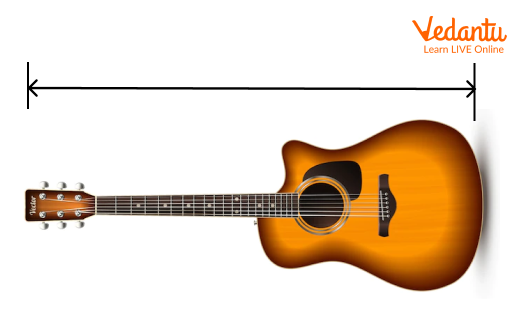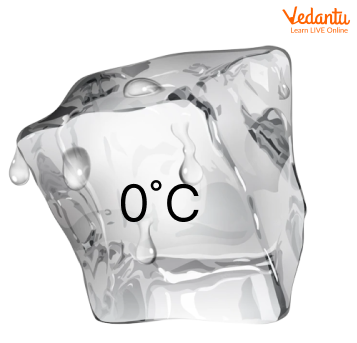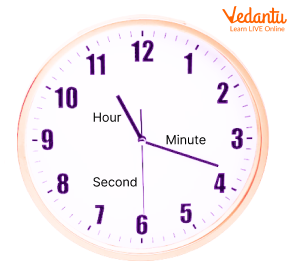Courses
Courses for Kids
Free study material
Free LIVE classes
More

# Let’s Understand Measurement in DetailLIVE
Join Vedantu’s FREE Mastercalss

## Measurement - A Method to Determine the Size or Amount of Something

You might have definitely heard the words - big, small, long, short etc. Do you know how we understand that a thing is long or short, big or small? For this calculation, we measure. Do you know what measurement is? It is nothing but a process of determining a number that represents the size or amount of something. Measurement plays a significant role in engineering, science, construction, other technical fields, and almost all other activities.

## What is the Measurement?

Measurement is defined as the act of determining the size of an object i.e., its length, weight, capacity, or other aspects. It can be better understood as a process of defining physical items using numbers. Let’s understand with an example:

## Measurement Example

Look at the 2 guitars below.Different lengths of Guitars

Here, we can say that the left guitar is smaller than the right guitar.

Although we are comparing two guitars here, we still don’t know the individual measurement of the two guitars. Hence, the statement “Left guitar is smaller than the right guitar” is serving a very limited purpose.

But, if we say the length of the left guitar is one metre whereas the length of the second guitar is 2 metres, therefore the first guitar is bigger than the second guitar by 1 metre.

This reason is giving us a better conclusion mathematically as here we know the measurement of individual guitars and their difference.

## Units of Measurement

The different units of measurement are:

Length

Length, also known as distance, measures the size of something from one end to the other.

Example of Length: The length of the guitar given here is one meter (slightly more than one yard).Length

Area

The area is the region occupied by a two-dimensional object such as a triangle, square, or circle, in a plane.

Example of Area: The dog is sitting inside one square meter tile.Area

Volume

Volume, also known as capacity, is defined as the amount of space occupied by a three-dimensional object.Volume

Here, the jug has exactly one litre or slightly more than 2 pints of water in it.

Mass (Weight)

Mass is defined as the amount of matter in any solid object or any volume of a liquid or gas.

Example of Mass: Here, the mass of a bar of gold is one kilogram or slightly more than 2 pounds.Mass (weight)

Temperature

Temperature defines how cold or hot an object is. The temperature is generally measured using the thermometer on a Celsius or Fahrenheit scale.

Example of Temperature: The block of ice here measures 0⁰ Celsius or 32⁰ Fahrenheit.Temperature

Time

Time is defined as the ongoing sequence of events taking place. The time is measured in seconds, minutes, hours, days, weeks, months, and years.

Example of Time: Here, the clock is showing at half past 6, or it can also be said as 6 hours 30 minutes.Time

System of Measurement

The two standard systems of measurement are:

• Metric System

• US Standard Units

Let’s briefly discuss the two measurements systems:

Metric System

The Metric System is the primary system of measurement. It is a measurement system that uses kilometres, litres, and milligrams to measure the length, volume, and weight of different objects. Also, the metric system is based on 10s. For example, a centigram is 10 times larger than the milligram and a litre is 10 times larger than the decilitre.

US Standard Units

The US standard unit is commonly used in the US. The different units of measurement in the US standard system include the following units:

Liquids: Fluid, Ounces, Cups, Pints, Quarts and Gallons

Mass: Pounds, Tons, and Ounces

Length: Feet, Inches, Miles, and Yards.

Temperature: Fahrenheit.

The US Standard Unit is also known as the United States Customary Units.

## Conclusion

In short, measurement is all about finding a number that represents the size or amount of something. Also, it is important to learn about measurement because it enables us to quantify the world around us.

Last updated date: 02nd Oct 2023
Total views: 133.5k
Views today: 2.33k

## FAQs on Let’s Understand Measurement in Detail

1. Why is the metric system of measurement also known as a decimal-based system?

The metric system of measurement is also known as the decimal-based system because it is based on the multiples of 10. Any measurement given in the unit such as Kilogram can be converted into another metric unit known as grams simply by moving the decimal point. For example, if the person weighs 75,200.0 grams, then this can be converted into kilograms simply by moving the decimal point three places to the left. In other words, the person weighs 75.2 kilograms.

2. What are the seven basic units of measurement?

The 7 basic units of measurement are Length — metre, Time — seconds, Temperature — kelvin, Electric Current —  ampere, Amount of substance — mole, Mass — Kilogram, and Luminous Intensity —  candela.

3. Why did the US not adopt the metric system of measurement?

The US did not adopt the metric system of measurement because of time and money. Also, it is quite time-consuming to translate between U.S. Customary System and the metric system.